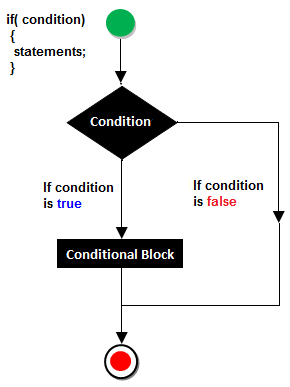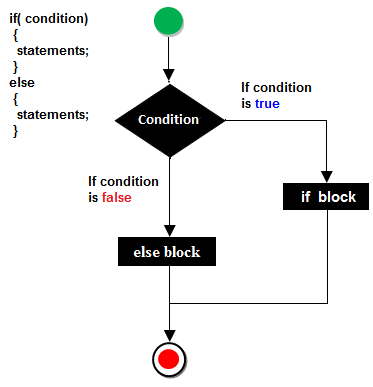Control Instructions in C

Program is a set of instructions. We know that each instruction of the program is executed by processor. Processor executes instructions one by one. What ever we write in our program will be executed in the same order as they appear in the program.

So we can say processor execute instructions in a sequential manner. At a particular instant processor is executing some line of code, we say control of processor is on that line. Processor’s control moves from one line to another. We say this movement of processor control goes in sequence.

Types of control instruction:

1. Sequence control instruction
2. Decision control instruction
3. Iterative control instruction
4. Switch case control instruction
5. goto instruction

Sequence control instruction:

Processor control moves in a sequential manner. We have to do nothing to implement sequence control instruction. This is just a concept that your program always run in a sequence.

Decision control instruction:

Decision control instruction is also known as selection control instruction. As the name implies the job is selection control instruction is to select a set of code for execution on the basis of some condition.

We can implement decision control instruction in three ways:

1. if
2. if-else
3. conditional operator (Ternary Operator)

C if statement

Syntax of if:

``````main()
{
..…
…..
if(some condition)
{
Statement1;
Statement2;
….
}
…
}
``````

if is a `keyword` which let compiler to identify decision control instruction. Immediately after if some condition is there.

This condition is any valid expression in C. If the result of expression is non-zero it is considered as TRUE otherwise FALSE

Immediately after this condition there is a block of code. Since this block is immediately after if, it is known as if block. Whatever we write in if block will be execute only when condition is TRUE. When condition is false control skip if block and execute statements written after if block.

Flowchart of if statement1. Constructing the body of `"if"` statement is always optional, Create the body when we are having multiple statements.
2. For a single statement, it is not required to specify the body.
3. If the body is not specified, then automatically condition part will be terminated with next semicolon `( ; )`.

### Example #1: C if statement

``````main()
{
int marks;
printf(“Enter marks: ”);
scanf(“%d”,&marks);
if(marks>=33)
{
printf(“You are PASS”);
}
if(marks<33)
{
printf(“You are FAIL”);
}
}
``````

Output 1

``````Enter marks:  45
You are PASS
``````

Output 2

``````Enter marks:  23
You are FAIL
``````

In this program output depends on the value given by user. Variable marks hold the value entered by user. We have used two `if `statements. In the first `if` statement we use the condition `marks>=33`, thus if the marks are greater than or equal to 33 condition becomes TRUE, so if block executed, otherwise if block is skipped.

Whatever may the result of first if condition, control has to reach second `if` statement. If marks are less than 33 condition will be TRUE and execute if block otherwise if block is skipped.

### Example #2: C if statement

``````// Program to display a number if user enters negative number
// If user enters positive number, that number won't be displayed

#include <stdio.h>
int main()
{
int number;

printf("Enter an integer: ");
scanf("%d", &number);

// Test expression is true if number is less than 0
if (number < 0)
{
printf("You entered %d.\n", number);
}

printf("The if statement is easy.");

return 0;
}

``````

Output 1

``````Enter an integer: -2
You entered -2.
The if statement is easy.``````

When user enters -2, the test expression `(number < 0)` becomes true. Hence, You entered -2 is displayed on the screen.

Output 2

``````Enter an integer: 5
The if statement in C programming is easy.
``````

When user enters 5, the test expression `(number < 0)` becomes false and the statement inside the body of `if` is skipped.

C if...else statement

The `if...else` statement executes some code if the test expression or some condition is true (nonzero) and some other code if the test expression or some condition is false (0).

OR

In general it can be used to execute one block of statement among two blocks, in C language `if` and `else` are the keyword in C.

Syntax of if...else

``````main()
{
..…
…..
if(some condition)
{
Statement1;
Statement2;
….
}
else
{
Statement1;
Statement2;
….
}
…
}
``````

If some condition is true, codes inside the body of `if` statement is executed and, codes inside the body of `else` statement is skipped.

If some condition is false, codes inside the body of `else` statement is executed and, codes inside the body of `if` statement is skipped.

Flowchart of if...else statementMIND IT !

You can use `if` statement without `else` block but `else` must have paired with `if`.

`Else` block should appear immediately after `if` block otherwise an error occurred during compilation.

### Example #1: C if...else statement

``````main()
{
int marks;
printf(“Enter marks ”);
scanf(“%d”,&marks);
if(marks>=33)
{
printf(“You are PASS”);
}
else
{
printf(“You are FAIL”);
}
}
``````

The same program was discussed using only `if` statements. This one is refined version of program and hence better than that of the previous one.

Notice that, there is only one condition need to be evaluated, if the condition is TRUE `if` block will work otherwise `else` block will be executed.

Note: If there is only one statement in `if` block then mentioning block using curly braces is optional. Same rule is applied to `else` block.

### Example #2: C if...else statement

``````// Program to check whether an integer entered by the user is odd or even
#include <stdio.h>
int main()
{
int number;
printf("Enter an integer: ");
scanf("%d",&number);

// True if remainder is 0
if( number%2 == 0 )
printf("%d is an even integer.",number);
else
printf("%d is an odd integer.",number);
return 0;
}
``````

Output

``````Enter an integer: 7
7 is an odd integer.
``````

When user enters 7, the test expression `( number%2 == 0 )` is evaluated to false. Hence, the statement inside the body of `else` statement `printf("%d is an odd integer");` is executed and the statement inside the body of `if` is skipped.

### Conditional operator ( ? :)

It is also known as ternary operator which means operator need three operands to perform its operation.

Syntax:

``````Expression1 ? expression2 : expression3;
``````

`Expression 1` is a condition, which is first evaluated as TRUE or FALSE. If the condition is TRUE executes `expression2` otherwise execute `expression3`.

Conditional operator works similar to `if-else`, but we do not have to use keyword `if` and `else`.

### Example #1: Conditional operator ( ? :)

``````main()
{
int x,y;
printf(“Enter two numbers:”);
scanf(“%d%d”,&x,&y);
x>y ? printf(“%d is greater”,x) : printf(“%d is greater”,y);
}
``````

Output

``````Enter two numbers: 45
33
45 is greater
``````

In this program user enters two numbers which is then get stored in x and y. Notice the last line of the program that is conditional operator, which is used to select one from the two `printf()` statements. If x>y then value of x get printed otherwise value of y is printed.

### Example #2: Selective assignment

``````main()
{
int x,y,max;
printf(“Enter two numbers”);
scanf(“%d%d”,&x,&y);
max=x>y ? x : y;
printf(“Greater number is %d”,max);
}
``````

Output

``````Enter two numbers: 85
100
Greater number is 100
``````

In this program conditional operator is used to select one from x and y to assign value of either x or y in variable max.